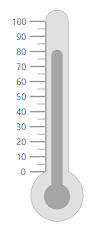Search results

# Appearance in Blazor Linear Gauge component

## Linear Gauge Area Customization

### Customize the Linear Gauge background

Using `Background` property and `LinearGaugeBorder` tag, you can change the background color and border of the Linear Gauge.

``````<SfLinearGauge Width ="200px" Height ="400px" Background= "skyblue">
<LinearGaugeBorder Color="#FF0000" Width="2">
</LinearGaugeBorder>
</SfLinearGauge>``````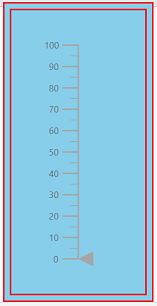## Linear Gauge Title

The title for the Linear Gauge can be set through `Title` property in `SfLinearGauge`. Its appearance can be customized using the `LinearGaugeTitleStyle`

``````<SfLinearGauge Title="Linear Gauge">
<LinearGaugeTitleStyle FontFamily="Arial"
FontWeight="regular"
FontStyle="italic"
Color="#E27F2D"
Size="23px">
</LinearGaugeTitleStyle>
</SfLinearGauge>``````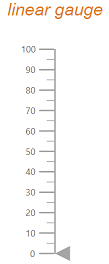## Linear Gauge Container

The area used to render the ranges and pointers at the center position of the gauge is called `Container`. It can be customized by using `Type`, `Offset`, `Width`, `Height` and `BackgroundColor` properties in `LinearGaugeContainer`. It is of three types namely,

• Normal
• Rounded Rectangle
• Thermometer

### Normal

The normal type will render the container as rectangle and this is the default container type.

``````<SfLinearGauge>
<LinearGaugeContainer Width="30">
<LinearGaugeAxes>
<LinearGaugeAxis>
<LinearGaugePointers>
<LinearGaugePointer Value="50" Width="15" Type="Point.Bar">
</LinearGaugePointer>
</LinearGaugePointers>
</LinearGaugeAxis>
</LinearGaugeAxes>
</LinearGaugeContainer>
</SfLinearGauge>``````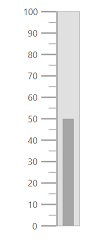### Rounded Rectangle

The rounded rectangle type will render the container as rectangle with rounded corners.

``````<SfLinearGauge>
<LinearGaugeContainer Width="30" Type="ContainerType.RoundedRectangle">
<LinearGaugeAxes>
<LinearGaugeAxis>
<LinearGaugePointers>
<LinearGaugePointer Value="50" Width="15" Type="Point.Bar">
</LinearGaugePointer>
</LinearGaugePointers>
</LinearGaugeAxis>
</LinearGaugeAxes>
</LinearGaugeContainer>
</SfLinearGauge>``````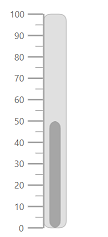### Thermometer

This type is used to render the container similar to the thermometer appearance.

``````<SfLinearGauge>
<LinearGaugeContainer Width="30" Type="ContainerType.Thermometer">
<LinearGaugeAxes>
<LinearGaugeAxis>
<LinearGaugePointers>
<LinearGaugePointer Value="80" Width="15" Type="Point.Bar">
</LinearGaugePointer>
</LinearGaugePointers>
</LinearGaugeAxis>
</LinearGaugeAxes>
</LinearGaugeContainer>
</SfLinearGauge>``````